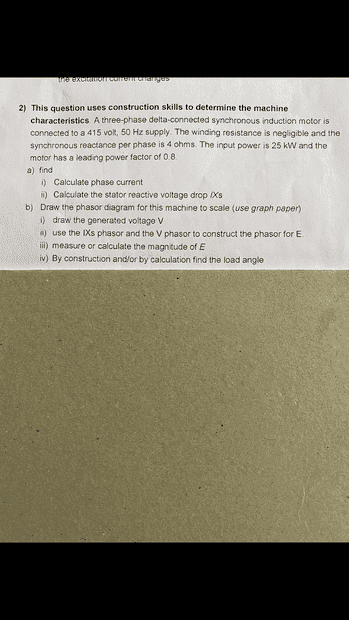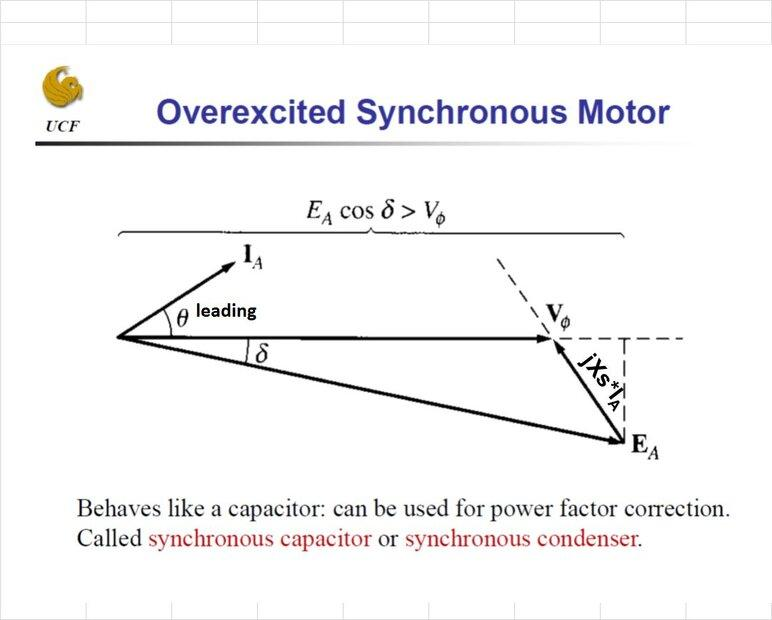# Phasor diagram help needed

Cassy85
Hi, I'm struggling to find out how to create a phasor diagram. I have attached a photo of the question any help would be greatly appreciated.This is what I have attempted so far.
It's question 2b I am really struggling with.
I'm not looking for the answers just looking for some direction.

1. Calculate phase current
Phase current = 250003 x 415 x 0.8
Phase current = 25.1 Amps
cos-10.8=36.87
I = 25.1 ∠36.87° Amps
1. Calculate the stator reactive voltage drop IXs

Voltage drop = IaXs
25.1 x 4 ∠36.87° + 90°
Voltage drop (IXs) = 100.4 ∠126.87° volts

First no such motor "synchronous induction motor" does exist .Since the power factor is leading it is about synchronous motor when the excitation current is more than rated.
The motor has an a.c. winding in stator part and a d.c. winding [poles] on the rotor. Since it is a separately E noted, the generated voltage is equal to supply voltage.
The generated voltage has to be in the load case.
If the power mentioned is "input power" the apparent power it is the ratio 25/0.8.The loaded current it is 25000/0.8/√3/415=43.475 A